# SpeedCalc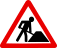This article or section is in the process of an expansion or major restructuring and is therefore classed as a work in progress. You are welcome to assist in its construction by editing it as well. If this article or section has not been edited in several days, please remove this template.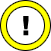This article is very short and not very detailed. Please help to improve it.
SpeedCalc
Developer Kevin Martian & Charles Brannon
Company ABC Publishing
Publisher Compute! Magazine
Release 1986
Licence Type-in Program
Platform C64, Apple II, & Atari
Operation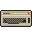Media Listing,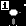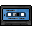Language(s)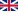Information Compute! January 1986 complete with source code.

SpeedCalc was the spreadsheet counterpart to the very popular SpeedScript word processor. Written by Kevin Martian and published by Compute! Magazine SpeedCalc offered very basic spreadsheet capability in a manner similar to VisiCalc spreadsheet developed for a in-home microcomputer.

Like SpeedScript, SpeedCalc was released as a type-in program. At 7.6 KByte long it was lengthy considering the manual input involved. In order to keep the file size down to a level the average reader would be willing to type, BASIC ROM math functions were leveraged extensively. In fact, only two SpeedCalc specific functions were created average and sum. The result was a program that was bare-bones even for the time, but still extremely flexible.

The cell types inferred by the user's input in a manner akin to duck typing. That is, unlike VisiCalc and similar spreadsheets SpeedCalc which required the user to declare the format of each cell before inputting data SpeedCalc infers the cell type based upon the input. Cells that do not conform to expected input patterns produce an *ERROR* cell.

Numbers. Numbers are values 1–9 expressed in base 10. Alternatively, scientific notation is supported as an input method but SpeedCalc converts these to standard base 10 for display purposes. Numbers may be positive or negative, thus a plus (+) or minus (-) may prefix a number and it is still valid. A decimal point is also optional but must be a period (.) and thousands separators are not supported. Because math routines are reused from BASIC, numbers incur the same limitations on decimal precision and range as they do in BASIC programs. Base 1 (binary), base 8 (octal) and base 16 (hexadecimal) are not supported number formats.
Formulas. Formulas must begin with an equals sign (=) and contain only supported mathematical operators, numbers (operands), cell references, parentheses, or built in functions.
Text. Text is any alpha numeric data that isn't a number or a formula. Thus 'Fifty Two' is text but so also is '10,000' due to the presence of the comma as a thousand's separator.

Only basic arithmetic operators were supported by SpeedCalc:

2. . Subtraction (-)
3. . Multiplication (*)
4. . Division (/)
5. . Exponentiation (↑)
6. . Equality (=)

SpeedCalc functions were taken directly from BASIC ROM. SpeedCalc's function names are the same as BASIC 2.0 keywords for the exact same function, except they are proceeded by the @ symbol and must be in lower case. The following is a list of SpeedCalc's available functions. For clarity, the OpenFormula equivalent function mnemonic is also supplied. Note that the two new SpeedCalc specific functions work on cell ranges only and do not take mathematical expressions as arguments.

SpeedCalc Function Codes
SpeedCalc Function OpenFormula Equivalent Notes
@abs() ABS() Returns the absolute value of a number.
@atn() ATAN() Returns the inverse trigonometric tangent of a number.
@ave() AVERAGE() Returns the average of the arguments. SpeedCalc's function expects a range of cells.
@cos() COS() Returns the cosine of the given angle (in radians).
@exp() EXP() Returns the mathematical constant e out to 8 decimal places raised to the power of a number.
@int() INT() Truncates a floating point number rounding down to the nearest integer.
@log() LOG() Returns the logarithm of a number to the specified base.
@sgn() SIGN() Returns the sign of a number. Returns 1 if the number is positive, -1 if negative and 0 if zero.
@sqr() SQRT() Returns the positive square root of a number.
@sin() SIN() Returns the sine of the given angle (in radians).
@sum() SUM() Adds all the numbers in a range of cells. SpeedCalc's function expects a range of cells.
@tan() TAN() Returns the tangent of the given angle (in radians).
@pi PI() Returns 3.14159265, the value of the mathematical constant π, out to 8 decimal places.

### Limitations

• Sheets are limited to 50 colums and 200 rows from AA:1 to BX:200.
• This gives a maximum sheet size of 50-columns × 200-rows = 10,000 cells total.
• Only about 10.5 KByte of RAM available for the spreadsheet, so it is not possible to populate more than about one third of a sheet's total cells.
• The input buffer is limited to 120 bytes (characters) the last of which must be RETURN (←).
• Advanced report formatting is only possible if the sheet is exported to SpeedScript and marked up from there.

## Operation

### SpeedScript Compatibility

Function Keys
Key Function
F1  Change boarder color.
F3  Change background color.
F5  Change text color.